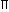## Search Here

### AREA AND VOLUME WORKOUT QUESTIONS

1.  A right triangle with sides 3 cm, 4 cm and 5 cm is rotated the side of 3 cm to form a cone. The volume of the cone so formed is:
 A. 12cm3 B. 15cm3 C. 16cm3 D. 20cm3

2.  In a shower, 5 cm of rain falls. The volume of water that falls on 1.5 hectares of ground is:
 A. 75 cu. m B. 750 cu. m C. 7500 cu. m D. 75000 cu. m

3.  A hall is 15 m long and 12 m broad. If the sum of the areas of the floor and the ceiling is equal to the sum of the areas of four walls, the volume of the hall is:
 A. 720 B. 900 C. 1200 D. 1800

4.  66 cubic centimeters of silver is drawn into a wire 1 mm in diameter. The length of the wire in meters will be:
 A. 84 B. 90 C. 168 D. 336

5.  A hollow iron pipe is 21 cm long and its external diameter is 8 cm. If the thickness of the pipe is 1 cm and iron weighs 8 g/cm3, then the weight of the pipe is:
 A. 3.6 kg B. 3.696 kg C. 36 kg D. 36.9 kg